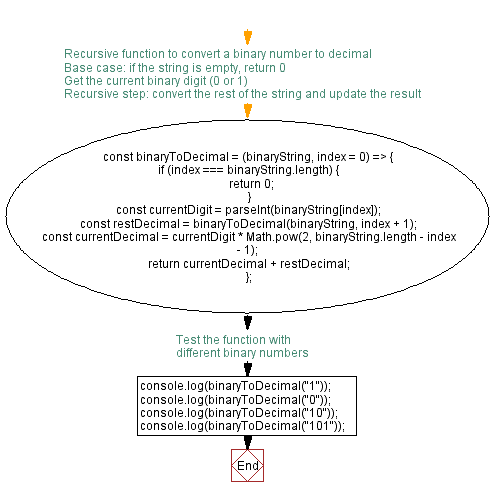# JavaScript: Convert Binary to Decimal using recursion

## JavaScript Function: Exercise-11 with Solution

Write a JavaScript program to convert binary number (positive) to decimal number using recursion.

Test Data:
(1) -> "1"
(0) -> "0"
(10) -> "1010"
(101) -> "1100101"

Sample Solution:

HTML Code:

``````<!DOCTYPE html>
<html>
<meta charset="utf-8">
<title>Convert Binary to Decimal using recursion</title>
<body>

</body>
</html>
```
```

JavaScript Code:

``````const test = (n) => {
if (n === 0 || n === 1)
{
return String(n)
}
return test(Math.floor(n / 2)) + String(n % 2)
}
console.log(test(1))
console.log(test(0))
console.log(test(10))
console.log(test(101))
```
```

Output:

```1
0
1010
1100101
```

Flowchart:Live Demo:

See the Pen javascript-recursion-function-exercise-11 by w3resource (@w3resource) on CodePen.

Improve this sample solution and post your code through Disqus

What is the difficulty level of this exercise?

Test your Programming skills with w3resource's quiz.

﻿